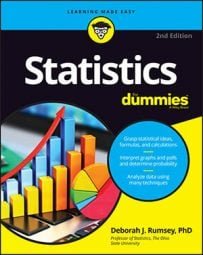##### Statistics For DummiesIf all you are interested in is where you stand compared to the rest of the herd, you need a statistic that reports relative standing, and that statistic is called a percentile. The kth percentile is a value in a data set that splits the data into two pieces: The lower piece contains k percent of the data, and the upper piece contains the rest of the data (which amounts to [100 – k] percent, because the total amount of data is 100 percent). Note: k is any number between 0 and 100.

The median is the 50th percentile: the point in the data where 50 percent of the data fall below that point, and 50 percent fall above it.

To calculate the kth percentile (where k is any number between 0 and 100), do the following steps:
1. Order all the values in the data set from smallest to largest.

2. Multiply k percent by the total number of values, n. If you have 10 pieces of data or values in the data set, n would equal 10.

The resulting number you get is called the index.

3. If the index obtained in Step 2 is not a whole number, round it up to the nearest whole number and go to Step 4. If the index obtained in Step 2 is a whole number, go to Step 5.

4. Count the values in your data set from left to right (from the smallest to the largest value) until you reach the number indicated by Step 3.

The corresponding value in your data set is the kth percentile.

5. Count the values in your data set from left to right until you reach the number indicated by Step 2 (the index).

The kth percentile is the average of that corresponding value in your data set and the value that directly follows it.

For example, suppose you have 25 test scores, and in order from lowest to highest they look like this: 43, 54, 56, 61, 62, 66, 68, 69, 69, 70, 71, 72, 77, 78, 79, 85, 87, 88, 89, 93, 95, 96, 98, 99, 99. To find the 90th percentile for these (ordered) scores, start by multiplying 90 percent times the total number of scores, which gives 90% ∗ 25 = 0.90 ∗ 25 = 22.5 (the index). Rounding up to the nearest whole number, you get 23.

Counting from left to right (from the smallest to the largest value in the data set), you go until you find the 23rd value in the data set. That value is 98, and it’s the 90th percentile for this data set.

Now say you want to find the 20th percentile. Start by taking 0.20 x 25 = 5 (the index); this is a whole number, so proceed from Step 3 to Step 4b, which tells you the 20th percentile is the average of the 5th and 6th values in the ordered data set (62 and 66). The 20th percentile then comes to (62 + 66) ÷ 2 = 64.

The median (the 50th percentile) for the test scores is the 13th score: 77.

The steps shown here demonstrate one way of calculating percentiles, but there are several other acceptable methods. Do not be too alarmed if your calculator or a friend gives you a value close to but different from what these steps would give.